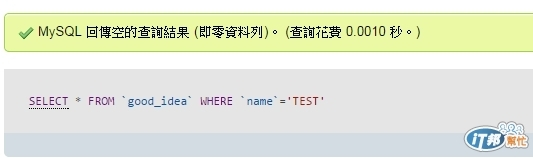#DAY 21
3

## [鐵人賽Day21]判斷資料庫回傳是否有資料(使用mysql_num_row來處理)

「mysql_nums.php」

``````<?php
\$input_name="TEST";

mysql_connect("127.0.0.1","root","");
mysql_select_db("ithome_test");
\$sql="SELECT * FROM `good_idea` WHERE `name`='\$input_name'";
\$result=mysql_query(\$sql);
while(\$row=mysql_fetch_array(\$result)){
\$db_name=\$row['name'];
}
if(\$input_name==\$db_name){
echo \$input_name."名稱已被使用";
}else{
echo \$input_name."名稱可以使用";
}
?>
``````「mysql_nums.php」

``````<?php
\$input_name="TEST";

mysql_connect("127.0.0.1","root","");
mysql_select_db("ithome_test");
\$sql="SELECT * FROM `good_idea` WHERE `name`='\$input_name'";
\$result=mysql_query(\$sql);
\$nums=mysql_num_rows(\$result);
if(\$nums > 0){
while(\$row=mysql_fetch_array(\$result)){
\$db_name=\$row['name'];
}
if(\$input_name==\$db_name){
echo \$input_name."名稱已被使用";
}
}else{
echo \$input_name."名稱可以使用";
}
?>
``````